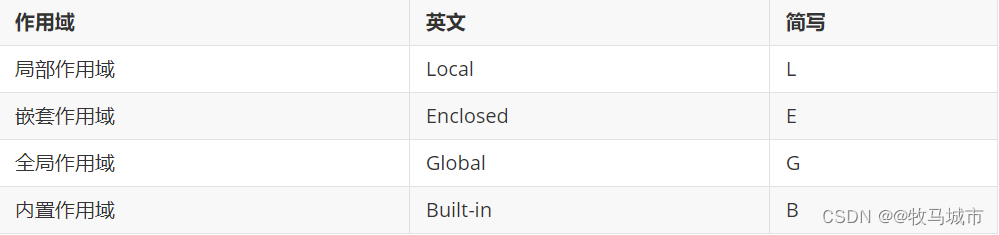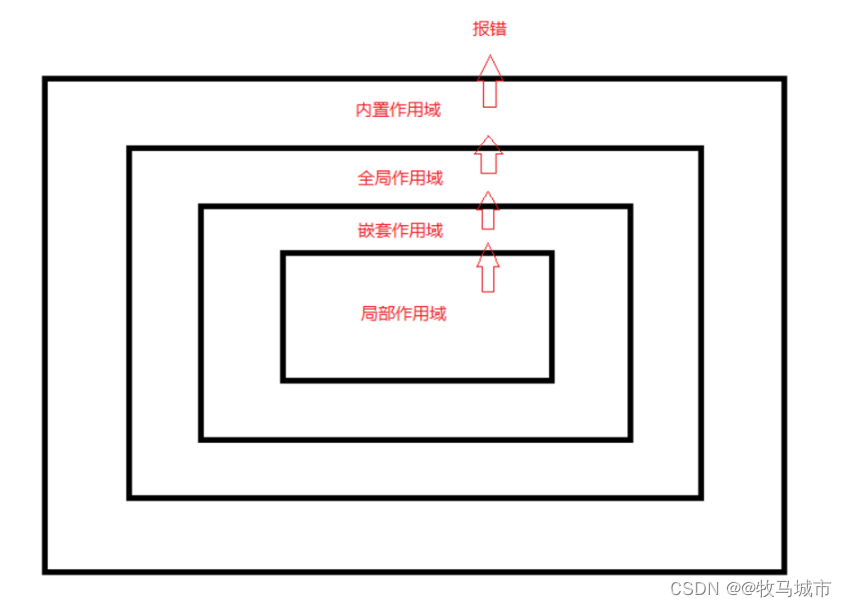## 作用域的类型

Python变量作用域可以分为四种，分别为局部作用域、嵌套作用域、全局作用域、内置作用域；四种作用域中变量的调用顺序采取“就近原则”，即 LEGB法则。1.局部作用域

``def func():    a = 0     # 局部变量    print("func a = ", a) a = 'Hello Python'  # a为全局变量func()print("函数外 a : ", a) 输出结果：func a = 0函数外 a : Hello Python``

2.嵌套作用域

``def func(i):    a = [1,2]    def func1():        a.append(i)        return a    return func1 cls = func(1)print(cls) 输出结果：[1,2,1]``

3.全局作用域

``a = 1     # a为全局变量 def func():    a = 2    # 局部变量    a += 1    print("局部变量 a = ",a) func()print("全局变量 a = ",a) 输出结果：局部变量 a = 3全局变量 a = 1``

``a = [1, 2, 3，4]b = {'语文': 100, "数学": 100} def func():    a.append(5)    b.update({"英语": 90})    print("函数中> a = ", a)    print("函数中> b = ", b)  func()print("函数外 a = ", a)print("函数外 b = ", b) 输出结果：函数中> a =  [1, 2, 3, 4，5]函数中> b =  {'语文': 100, '数学': 100, '英语': 90}函数外 a =  [1, 2, 3, 4，5]函数外 b =  {'语文': 100, '数学': 100, '英语': 90}``

``a = 1 def func():    global a     # 在使用a之前用global    a += 1    print("函数中> a = ", a) func()print("函数外 a = ", a) 输出结果：函数中> a =  2函数外 a =  2``

4.内置作用域

## 层级关系

python的四种所用域及其调用顺序，当使用某一变量时，首先在函数内部进行搜索（局部作用域 L），搜索不到便到上层函数或类中进行查找（嵌套作用域 E），再查找不到便到更上层模块中进行查找（全局作用域 G）,还查找不到则进入预先定义的（内置作用域 B）中查找，查找不到则报错。